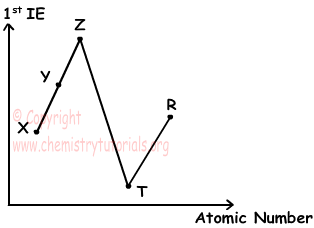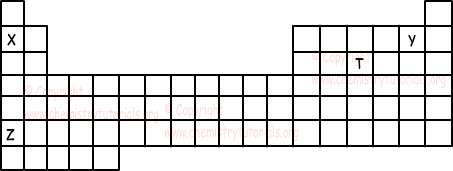## Periodic Table Exam2 and Problem Solutions

Periodic Table Exam2 and  Problem Solutions

1. First ionization energies vs. atomic mass graph of X, Y, Z, T and R is given below.If Z is in 3rd period, which one of the following statements is false?

I. Atomic number of X is 16

II. Y is in p block

III. Z and T are in same period

IV. Electron configuration of R shows spherical symmetry property

Solution:

Since change in the first ionization energy of Z and T are too high, Z is noble gas and T is in I A group. Thus, Z is noble gas in 3rd period and it has following electron configuration;

Z: 1s22s22p63s23p6

Atomic number of Z is 18

I. Atomic number of X = Atomic number of Z - 2

Atomic number of X is 16. I is true

II. Y has atomic number 17 and has following electron configuration;

Y: 1s22s22p63s23p5

As you can see Y is in p block. II is true.

III. Z is in 3rd period but T is in fourth period. III is false.

IV. Electron configuration of R is given below;

Z: 1s22s22p63s23p64s2

Since all orbitals are filed, R shows spherical symmetry property. IV is true.

2. Which one of the following statements is false for elements given in the periodic table?I. Electron affinity of Y is larger than others

II. X and T share electrons and form compound.

III. Z has largest atomic radius

IV. Ionic property of ZY compound is larger than others

Solution:

I. Electron affinity increases as we move from left to right and top to bottom in periodic table. Thus, Y has larger electron affinity, I is true.

II. Since X is in I A group, it is metal and T is in VA and nonmetal. So, X and T can form compound by electron transfer, not by electron sharing. II is false.

III. Atomic radii increases as we move from right to left and top to bottom in periodic table. So, Z has largest atomic radii, III is true.

IV. Increasing in the electronegativity increases ionic property of compounds. Electronegativity increases from left to right and decreases from top to bottom in periodic table. Z is the one having smallest electronegativity and K is the one having highest electronegativity. Difference between electronegativities of these elements make compound have largest ionic property. IV is true.

3. Which ones of the following statements are true for 4X, 9Y, 17Z?

I. X and Z are in same group elements

II. X and Y are same period elements

III. X and Z form ionic compound

Solution:

We first write electron configuration of elements to find group and period numbers.

4X: 1s22s2

9Y: 1s22s22p5

17Z: 1s22s22p63s23p5

I. Number of electrons in last shell orbitals give group number;

Y and Z are in VII A group. I is true

II. Last shell number gives period number. So, X and Y are in second period. II is true.

III. Since X is in II A group it is metal and Y is in VII A group and it is nonmetal. Property of compound is ionic. III is true.

4. Which ones of the following statements are always true related to periodic table?

I. s and d block elements are all metal

II. p block elements are nonmetal and noble gases

III. There are nonmetals before noble gases.

IV. There are halogens before noble gases

Solution:

H is in I A group but it is not metal. I is false.

p block elements are metals, nonmetals and noble gases. II is false

When we examine periodic table we see that there are always nonmetals before noble gases. III is true.

H comes before He and it is not halogen. Thus, IV is false.

5. Electron configuration of X+2 last with 2p6. Which one of the following elements have similar chemical properties with X element?

I. 8Y

II. 20Z

III. 4T

Solution:

Electron configuration of X+2 ion;

X+2: 1s22s22p6 (X gives 2 electrons)

X: 1s22s22p63s2 ( X is in II A group)

Elements in same groups show similar chemical properties. Now we find group numbers of given elements;

8Y: 1s22s22p4 (Y is in VI A group)

20Z: 1s22s22p63s23p64s2 (Z is in II A group)

4T: 1s22s2 (T is in II A group)

Z and T are in same group with X, thus they have similar chemical properties.

Related